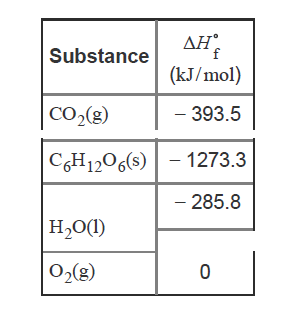# Problem: Calculate the mass of glucose metabolized by an 89.5 kg person in climbing a mountain with an elevation gain of 1350 m. Assume that the work performed in the climb is four times that required to simply lift 89.5 kg by 1350 m.The metabolism of glucose, C6H12O6, yields carbon dioxide, CO2(g), and water, H2O(l), as products. Energy released in this metabolic process is converted to useful work, w, with about 66.0% efficiency. The formula for work isw = mdgwhere m is mass, d is distance, and g = 9.81 m ⋅ s−2 is the acceleration due to gravity.Use the data below to answer questions about the metabolism of glucose.

###### FREE Expert Solution
98% (261 ratings)View Complete Written Solution
###### Problem Details

Calculate the mass of glucose metabolized by an 89.5 kg person in climbing a mountain with an elevation gain of 1350 m. Assume that the work performed in the climb is four times that required to simply lift 89.5 kg by 1350 m.

The metabolism of glucose, C6H12O6, yields carbon dioxide, CO2(g), and water, H2O(l), as products. Energy released in this metabolic process is converted to useful work, w, with about 66.0% efficiency. The formula for work is

w = mdg

where m is mass, d is distance, and g = 9.81 m ⋅ s−2 is the acceleration due to gravity.

Use the data below to answer questions about the metabolism of glucose.